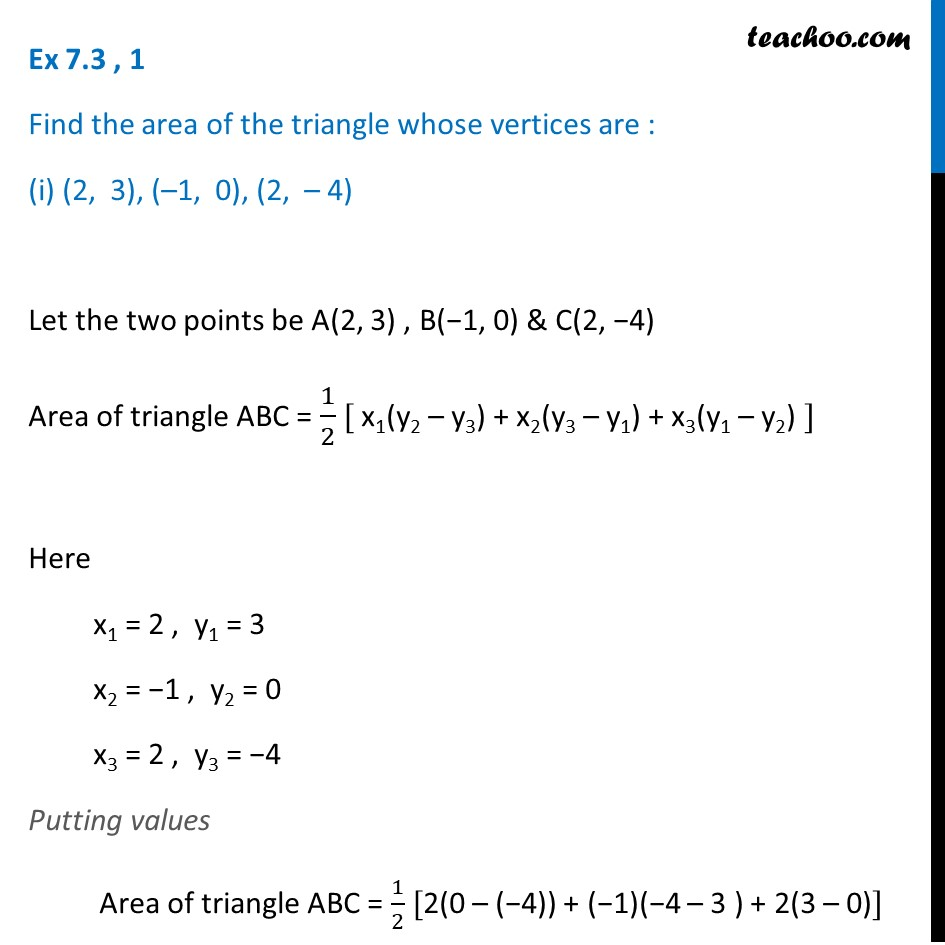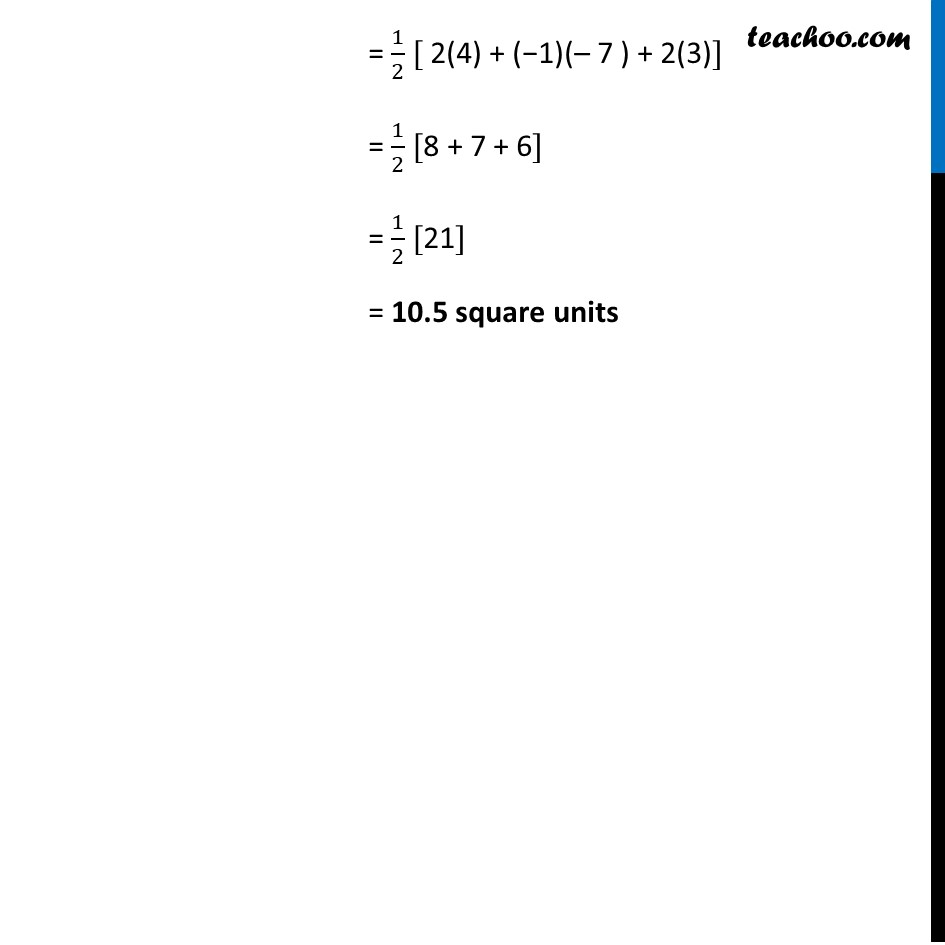Chapter 7 Class 10 Coordinate Geometry

Class 10
Important Questions for Exam - Class 10Introducing your new favourite teacher - Teachoo Black, at only ₹83 per month

### Transcript

Ex 7.3 , 1 Find the area of the triangle whose vertices are : (i) (2, 3), (–1, 0), (2, – 4) Let the two points be A(2, 3) , B(−1, 0) & C(2, −4) Area of triangle ABC = 1/2 [ x1(y2 – y3) + x2(y3 – y1) + x3(y1 – y2) ] Here x1 = 2 , y1 = 3 x2 = −1 , y2 = 0 x3 = 2 , y3 = −4 Putting values Area of triangle ABC = 1/2 [2(0 – (−4)) + (−1)(−4 – 3 ) + 2(3 – 0)] = 1/2 [ 2(4) + (−1)(– 7 ) + 2(3)] = 1/2 [8 + 7 + 6] = 1/2  = 10.5 square units